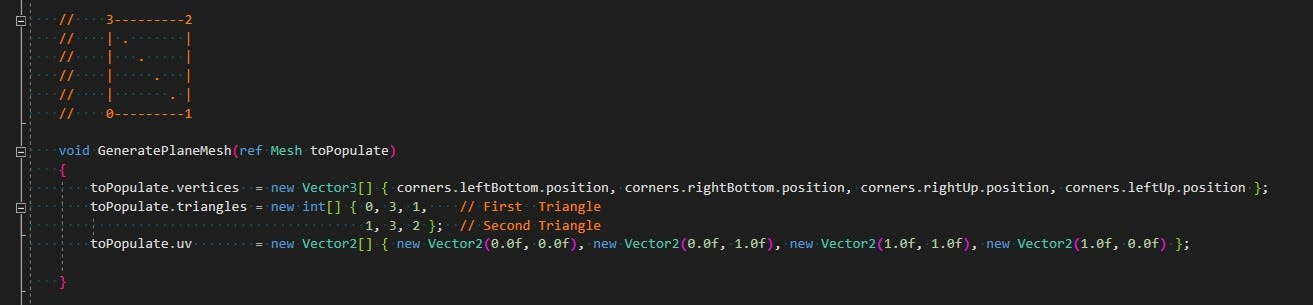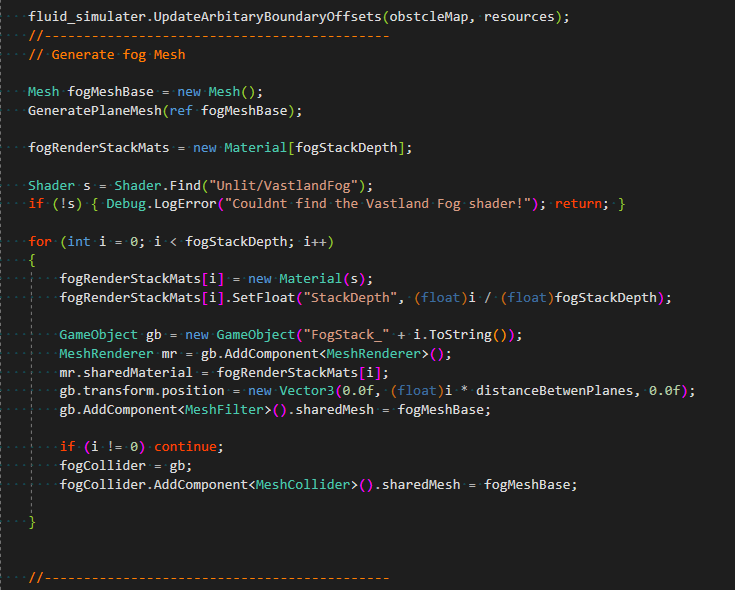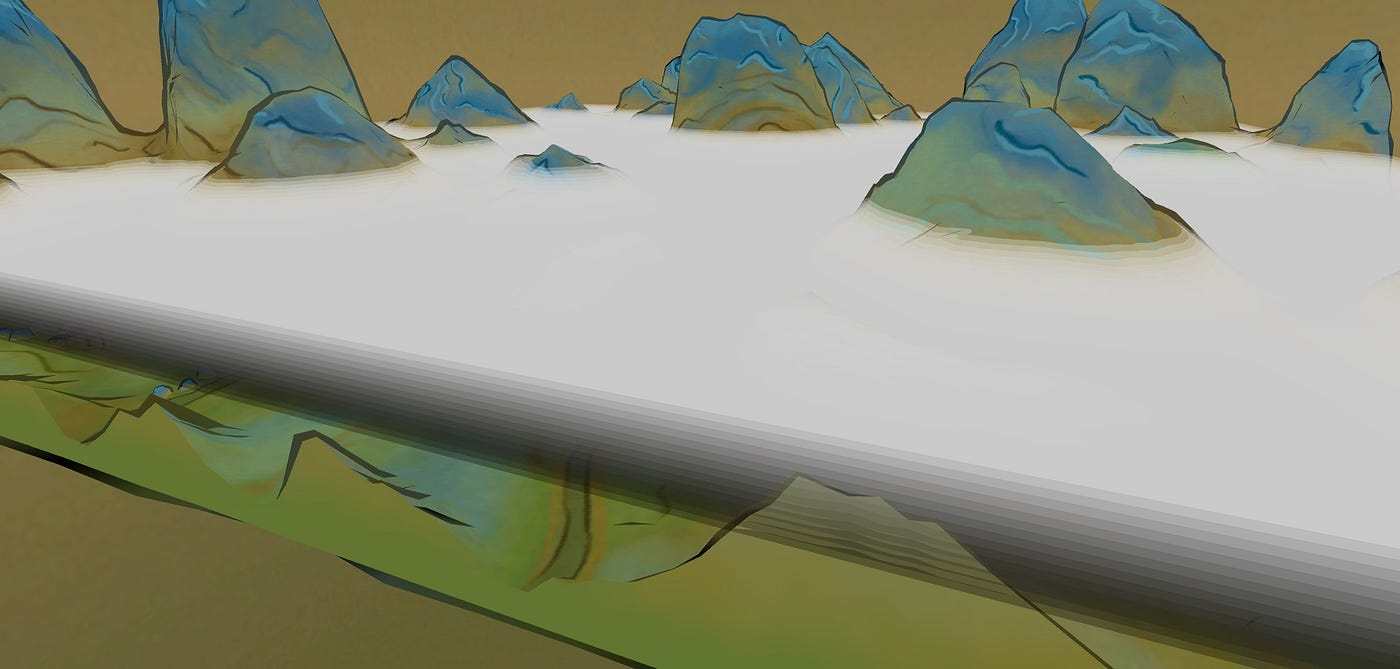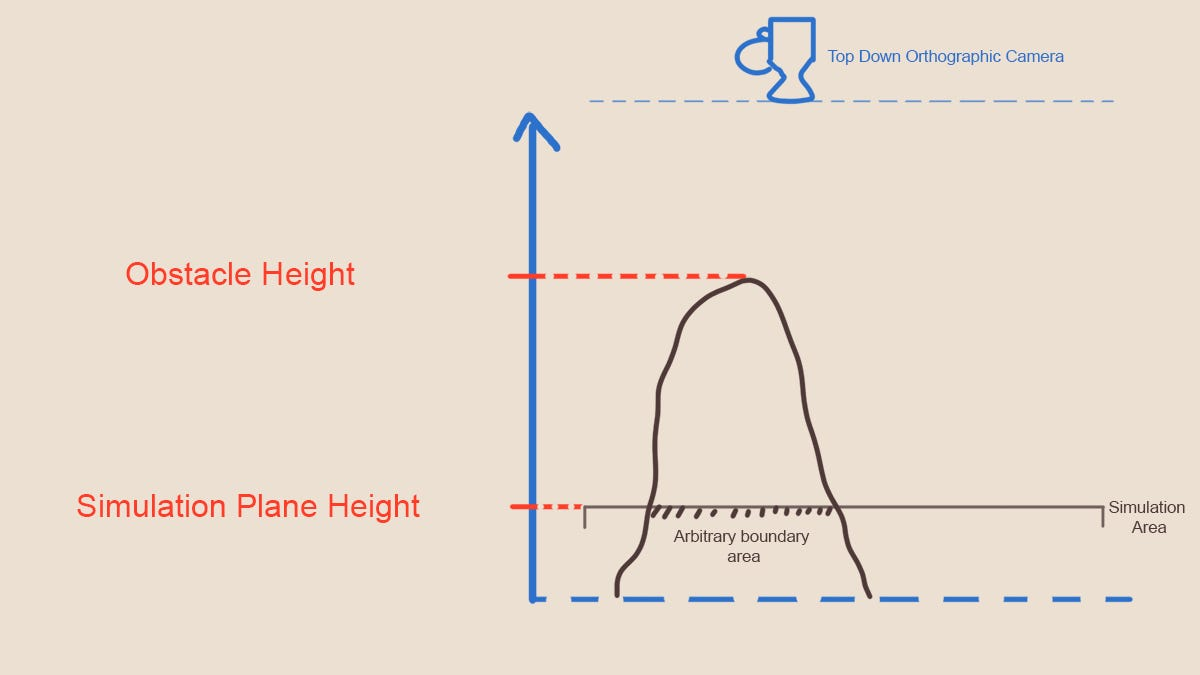# Rendering VolumetricFigure -1- Code for generating a single plane meshFigure -2- Creating the stack of layersFigure -3- The stacks visualization

# Creating a Obstacle MapFigure -4- Comparing the Height of obstacles with simulation area height in camera space
1. Render the depth of the scene (all objects that should act as obstacles). The camera should be orthogonal to the simulation space with an orthogonal projection (in our case and the usual case this would be a top down view, where the camera is looking at the simulation)
2. Take the texture produced in step 1 and run a check to see which pixel is closer to the camera than the simulation plane (hence is higher than the simulation plane). Mark those pixels as white, which encodes a pixel covered by obstacles.
3. After step 2, you are left with a black and white mask of all the obstacles which you provide the fluid engine.

## Creating a Depth Map for the Obstacles

1. Model (local space) to world space: local2World Matrix
2. World space to Camera (local space of camera game object) space: View Matrix
3. Camera space to Clip Space: Projection Matrix
1. I take the vectors which represents the edges of the simulation quad, and by using the cross product find the normal of the simulation plane.
2. Then I find the middle point of the simulation area, and add the normal times an offset to the mid point, this way we place the camera somewhere above the simulation space.
3. For the forward vector of the camera we take the flipped normal of the plane (-normal), for the right vector one of the edges of the simulation quad (preferably the one along the x axis) and for up we take the cross product between the two.